# Lecture16 5

13. Apr 2015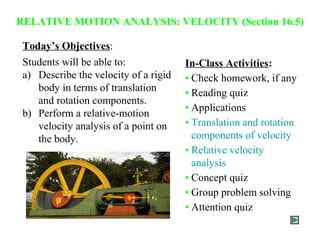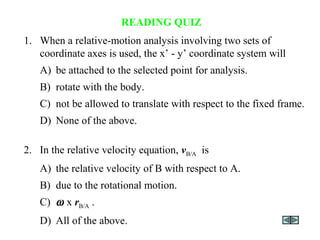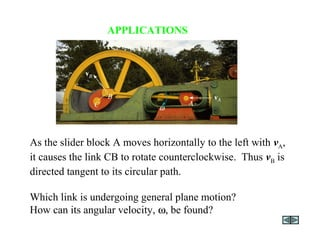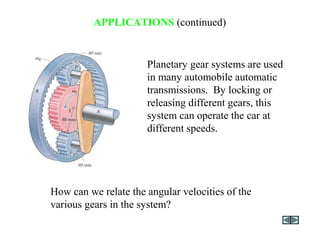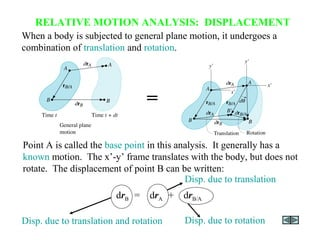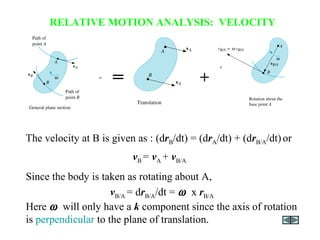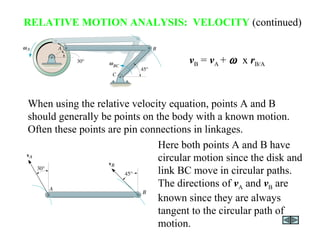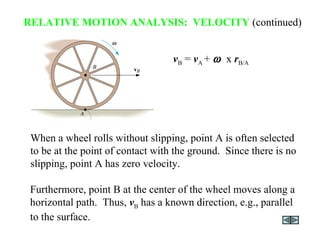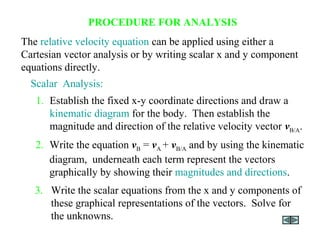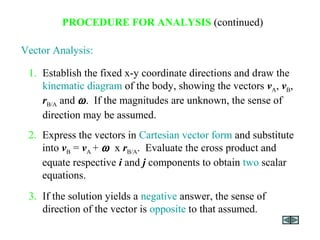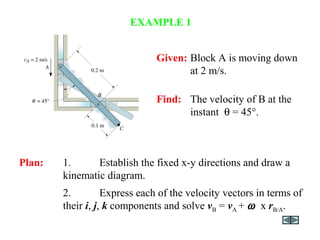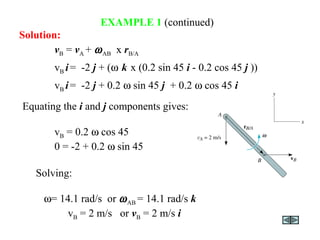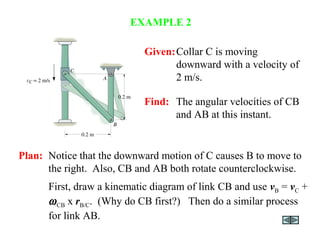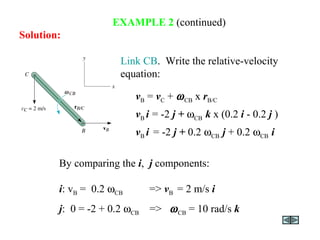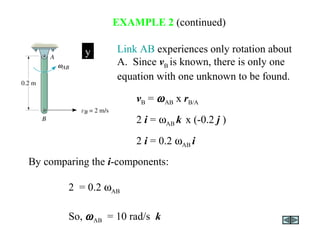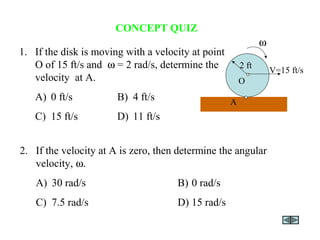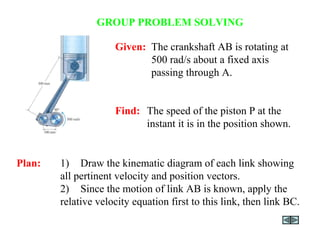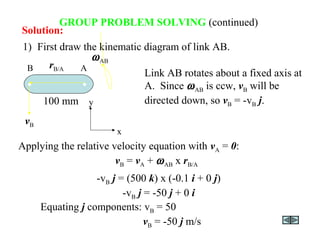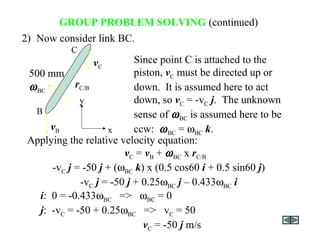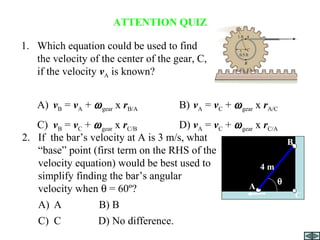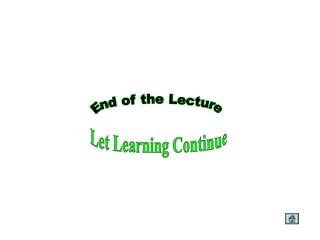1 von 21

### Lecture16 5

• 1. Today’s Objectives: Students will be able to: a) Describe the velocity of a rigid body in terms of translation and rotation components. b) Perform a relative-motion velocity analysis of a point on the body. RELATIVE MOTION ANALYSIS: VELOCITY (Section 16.5) In-Class Activities: • Check homework, if any • Reading quiz • Applications • Translation and rotation components of velocity • Relative velocity analysis • Concept quiz • Group problem solving • Attention quiz
• 2. READING QUIZ 1. When a relative-motion analysis involving two sets of coordinate axes is used, the x’ - y’ coordinate system will A) be attached to the selected point for analysis. B) rotate with the body. C) not be allowed to translate with respect to the fixed frame. D) None of the above. 2. In the relative velocity equation, vB/A is A) the relative velocity of B with respect to A. B) due to the rotational motion. C) ω x rB/A . D) All of the above.
• 3. APPLICATIONS As the slider block A moves horizontally to the left with vA, it causes the link CB to rotate counterclockwise. Thus vB is directed tangent to its circular path. Which link is undergoing general plane motion? How can its angular velocity, ω, be found?
• 4. APPLICATIONS (continued) Planetary gear systems are used in many automobile automatic transmissions. By locking or releasing different gears, this system can operate the car at different speeds. How can we relate the angular velocities of the various gears in the system?
• 5. RELATIVE MOTION ANALYSIS: DISPLACEMENT When a body is subjected to general plane motion, it undergoes a combination of translation and rotation. = drB = drA + drB/A Disp. due to translation and rotation Disp. due to translation Disp. due to rotation Point A is called the base point in this analysis. It generally has a known motion. The x’-y’ frame translates with the body, but does not rotate. The displacement of point B can be written:
• 6. The velocity at B is given as : (drB/dt) = (drA/dt) + (drB/A/dt)or vB = vA + vB/A RELATIVE MOTION ANALYSIS: VELOCITY = + Since the body is taken as rotating about A, vB/A = drB/A/dt = ω x rB/A Here ω will only have a k component since the axis of rotation is perpendicular to the plane of translation.
• 7. When using the relative velocity equation, points A and B should generally be points on the body with a known motion. Often these points are pin connections in linkages. Here both points A and B have circular motion since the disk and link BC move in circular paths. The directions of vA and vB are known since they are always tangent to the circular path of motion. vB = vA + ω x rB/A RELATIVE MOTION ANALYSIS: VELOCITY (continued)
• 8. Furthermore, point B at the center of the wheel moves along a horizontal path. Thus, vB has a known direction, e.g., parallel to the surface. vB = vA + ω x rB/A RELATIVE MOTION ANALYSIS: VELOCITY (continued) When a wheel rolls without slipping, point A is often selected to be at the point of contact with the ground. Since there is no slipping, point A has zero velocity.
• 9. PROCEDURE FOR ANALYSIS 3. Write the scalar equations from the x and y components of these graphical representations of the vectors. Solve for the unknowns. 1. Establish the fixed x-y coordinate directions and draw a kinematic diagram for the body. Then establish the magnitude and direction of the relative velocity vector vB/A. Scalar Analysis: 2. Write the equation vB = vA + vB/A and by using the kinematic diagram, underneath each term represent the vectors graphically by showing their magnitudes and directions. The relative velocity equation can be applied using either a Cartesian vector analysis or by writing scalar x and y component equations directly.
• 10. Vector Analysis: 3. If the solution yields a negative answer, the sense of direction of the vector is opposite to that assumed. 2. Express the vectors in Cartesian vector form and substitute into vB = vA + ω x rB/A. Evaluate the cross product and equate respective i and j components to obtain two scalar equations. 1. Establish the fixed x-y coordinate directions and draw the kinematic diagram of the body, showing the vectors vA, vB, rB/A and ω. If the magnitudes are unknown, the sense of direction may be assumed. PROCEDURE FOR ANALYSIS (continued)
• 11. Given: Block A is moving down at 2 m/s. Find: The velocity of B at the instant θ = 45°. Plan: 1. Establish the fixed x-y directions and draw a kinematic diagram. 2. Express each of the velocity vectors in terms of their i, j, k components and solve vB = vA + ω x rB/A. EXAMPLE 1
• 12. EXAMPLE 1 (continued) Equating the i and j components gives: vB = 0.2 ω cos 45 0 = -2 + 0.2 ω sin 45 vB = vA + ωAB x rB/A vB i= -2 j + (ω k x (0.2 sin 45 i - 0.2 cos 45 j )) vB i= -2 j + 0.2 ω sin 45 j + 0.2 ω cos 45 i Solution: Solving: ω = 14.1 rad/s or ωAB = 14.1 rad/s k vB = 2 m/s or vB = 2 m/s i
• 13. Given:Collar C is moving downward with a velocity of 2 m/s. Find: The angular velocities of CB and AB at this instant. Plan: Notice that the downward motion of C causes B to move to the right. Also, CB and AB both rotate counterclockwise. First, draw a kinematic diagram of link CB and use vB = vC + ωCB x rB/C. (Why do CB first?) Then do a similar process for link AB. EXAMPLE 2
• 14. EXAMPLE 2 (continued) Link CB. Write the relative-velocity equation: By comparing the i, j components: i: vB = 0.2 ωCB => vB = 2 m/s i j: 0 = -2 + 0.2 ωCB => ωCB = 10 rad/s k vB = vC + ωCB x rB/C vB i = -2 j + ωCB k x (0.2 i - 0.2 j ) vB i = -2 j + 0.2 ωCB j + 0.2 ωCB i Solution:
• 15. EXAMPLE 2 (continued) Link AB experiences only rotation about A. Since vB is known, there is only one equation with one unknown to be found. By comparing the i-components: 2 = 0.2 ωAB So, ωAB = 10 rad/s k x y vB = ωAB x rB/A 2 i = ωAB k x (-0.2 j ) 2 i = 0.2 ωAB i
• 16. CONCEPT QUIZ 1. If the disk is moving with a velocity at point O of 15 ft/s and ω = 2 rad/s, determine the velocity at A. A) 0 ft/s B) 4 ft/s C) 15 ft/s D) 11 ft/s 2. If the velocity at A is zero, then determine the angular velocity, ω. A) 30 rad/s B) 0 rad/s C) 7.5 rad/s D) 15 rad/s 2 ft V=15 ft/s ω A O
• 17. GROUP PROBLEM SOLVING Given: The crankshaft AB is rotating at 500 rad/s about a fixed axis passing through A. Find: The speed of the piston P at the instant it is in the position shown. Plan: 1) Draw the kinematic diagram of each link showing all pertinent velocity and position vectors. 2) Since the motion of link AB is known, apply the relative velocity equation first to this link, then link BC.
• 18. GROUP PROBLEM SOLVING (continued) Solution: -vB j = (500 k) x (-0.1 i + 0 j) -vB j = -50 j + 0 i 1) First draw the kinematic diagram of link AB. Link AB rotates about a fixed axis at A. Since ωAB is ccw, vB will be directed down, so vB = -vB j. Equating j components: vB = 50 vB = -50 j m/s Applying the relative velocity equation with vA = 0: vB = vA + ωAB x rB/A ωAB y x 100 mm rB/A vB B A • •
• 19. GROUP PROBLEM SOLVING (continued) -vC j = -50 j + (ωBC k) x (0.5 cos60 i + 0.5 sin60 j) -vC j = -50 j + 0.25ωBC j – 0.433ωBC i 2) Now consider link BC. Since point C is attached to the piston, vC must be directed up or down. It is assumed here to act down, so vC = -vC j. The unknown sense of ωBC is assumed here to be ccw: ωBC = ωBC k. i: 0 = -0.433ωBC => ωBC = 0 j: -vC = -50 + 0.25ωBC => vC = 50 vC = -50 j m/s Applying the relative velocity equation: vC = vB + ωBC x rC/B y x 500 mm rC/B vB ωBC B C vC
• 20. ATTENTION QUIZ 1. Which equation could be used to find the velocity of the center of the gear, C, if the velocity vA is known? A) vB = vA + ωgear x rB/A B) vA = vC + ωgear x rA/C C) vB = vC + ωgear x rC/B D) vA = vC + ωgear x rC/A vA 2. If the bar’s velocity at A is 3 m/s, what “base” point (first term on the RHS of the velocity equation) would be best used to simplify finding the bar’s angular velocity when θ = 60º? A) A B) B C) C D) No difference. A 4 m B θ C

### Hinweis der Redaktion

1. Answers: A. D B. C
2. Answers: 1. B 2. A